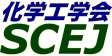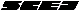## \$B9V1i%W%m%0%i%`!J2q>l!&F|DxJL!K(B

### L\$B2q>l(B \$BBh(B3\$BF|(B

\$B:G=*99?7F|;~!'(B2012-08-20 15:02:17
\$B9V1i(B
\$B;~9o(B
\$B9V1i(B
\$BHV9f(B
\$B9V1iBjL\!?H/I=\$B%-!<%o!<%I(B\$BJ,N`(B
\$BHV9f(B
\$B\$BHV9f(B
\$B%7%s%]%8%&%`(B \$B!c
(8:40\$B!A(B10:00)\$B!!(B(\$B:BD9(B \$B2O@%(B \$B85L@(B)
8:40\$B!A(B 9:00L300\$B%a%?%N!<%k%*!<%H%5!<%^%k2~CHq\$K4X\$9\$k8&5f(B
(\$B7DXfBg(B) \$B!{(B(\$B3X(B)\$BBgC+(B \$B=a(B \$B!&(B \$BOBED(B \$B>;>M(B \$B!&(B M. F. Hasan \$B!&(B (\$B@5(B)\$B2#?9(B \$B9d(B \$B!&(B (\$B@5(B)\$B?"ED(B \$BMx5W(B
Reforming
Hydrogen
Auto-Thermal
S-1224
9:00\$B!A(B 9:20L301\$B%*%U%,%9:F=[4D\$rH<\$&%*!<%H%5!<%^%k2~
(\$B7DXfBgM}9)(B) \$B!{(B(\$B3X(B)\$B;~G\$(B \$B:L(B \$B!&(B (\$B3X(B)\$B@uLn(B \$B;J(B \$B!&(B (\$B3X(B)Hasan M. F. \$B!&(B (\$B@5(B)\$B2#?9(B \$B9d(B \$B!&(B (\$B@5(B)\$B?"ED(B \$BMx5W(B
reformer
autothermal
off-gas recirculation
S-1237
9:20\$B!A(B 9:40L302\$BDLEE2CG.%"%k%^%\$%H?(G^\$rMQ\$\$\$?%a%?%s?e>x5\$2~
(\$BElG@9)Bg(B) \$B!{(B(\$B3X(B)\$B1|Ln(B \$B@i1{(B \$B!&(B (\$B3X(B)\$BD%(B \$B7D51(B \$B!&(B (\$B@5(B)\$B3T(B \$B%f(B \$B!&(B (\$B@5(B)\$B55;3(B \$B=(M:(B \$B!&(B (\$B@5(B)\$B:y0f(B \$B@?(B
Steam reforming
Anodic alumina support
Nickel catalyst
S-1389
9:40\$B!A(B 10:00L303\$B%j%s;@7AG3NAEECS%7%9%F%`\$N;@2=H?1~4o\$H\$7\$F\$N1~MQ(B
(\$BL>Bg1!9)(B) \$B!{(B(\$B3X(B)\$B2->e(B \$B?? \$B!&(B (\$B@5(B)\$B;3ED(B \$BGn;K(B \$B!&(B (\$B@5(B)\$BED@n(B \$BCRI'(B \$B!&(B (\$BL>;T9)8&(B) (\$B@5(B)\$B5\ED(B \$B9/;K(B
Fuel cell reactor
PAFC
Selecteve oxidation
S-1840
(10:00\$B!A(B11:00)\$B!!(B(\$B:BD9(B \$B8E;3(B \$BDL5W(B)
10:00\$B!A(B 10:20L304\$BDX303L\$r86NA\$H\$9\$k%a%=9&C:AG\$ND4@=\$H%-%c%Q%7%?FC@-(B
(\$B:eI\Bg1!(B) \$B!{(B(\$B3X(B)\$BBgC+(B \$B=S2p(B \$B!&(B (\$B@5(B)\$BIpF#(B \$BL@FA(B
capacitor
mesopore
carbon
S-1675
10:20\$B!A(B 10:40L305\$B%0%i%U%'%s\$NMO1U9g@.!"%J%N9=B\$@)8f\$HBgMFNL%-%c%Q%7%?\$X\$N1~MQ(B
(\$BElKLBgB?858&(B) \$B!{;0C+(B \$BM!(B \$B!&(B \$B%^%i%Q%s(B \$B%5%F%#%C%7%e(B \$B!&(B \$B1':,K\(B \$BFF(B \$B!&(B \$Bcx5o(B \$B9bL@(B \$B!&(B (\$B@5(B)\$BK\4V(B \$B3J(B
Graphene
Capacitor
Electrode
S-1466
10:40\$B!A(B 11:00L306\$B%^%\$%/%m%A%c%M%k\$r7A@.\$7\$?9=B\$2=EE6K\$K\$h\$kEE5\$Fs=EAX%-%c%Q%7%?\$NB.EY@-G=8~>e(B
(\$B5~Bg9)(B) \$B!{(B(\$B3X(B)\$BBgAR(B \$B:M>:(B \$B!&(B (\$B3X(B)\$B \$B!&(B (\$B@5(B)\$B0f>e(B \$B85(B \$B!&(B (\$B@5(B)\$B2O@%(B \$B85L@(B
Electrochemical double-layer capacitor
Structured electrode
High discharge rate
S-183
(11:00\$B!A(B12:00)\$B!!(B(\$B:BD9(B \$B0f>e(B \$B85(B)
11:00\$B!A(B 11:20L307\$BHyJ4%;%k%m!<%9!A%]%j%"%/%j%k%K%H%j%kJ#9gA!0]\$NC:AG:`NA2=\$H%j%A%&%`%\$%*%s%-%c%Q%7%?\$X\$NE,MQ(B
(\$B:eI\Bg1!9)(B) \$B!{(B(\$B3X(B)\$B?eLn(B \$B5.A1(B \$B!&(B (\$B@5(B)\$BIpF#(B \$BL@FA(B \$B!&(B (\$B2,;3Bg(B) \$B2-86(B \$B9*(B \$B!&(B \$BLZ2<(B \$B72Kc(B \$B!&(B (\$BF|K\%(%/%9%i%s(B) \$BBgOB(B \$B2B5V(B
lithium ion capacitor
polyacrylonitrile
cellulose
S-1284
11:20\$B!A(B 11:40L308\$B%j%A%&%`%\$%*%sEECS\$K\$*\$1\$k3hJ*
(\$B6bBtBg1!<+(B) \$B!{(B(\$B3X(B)\$BC#@n(B \$B1Q<#(B \$B!&(B \$BCSED(B \$BCR=((B \$B!&(B (\$B6bBtBgM}9)(B) (\$B@5(B)\$BEDB<(B \$BOB90(B
Lithium-ion battery
Intercalation electrode
Diffusion process
S-1898
11:40\$B!A(B 12:00L309\$BL5MOG^H?1~\$rMQ\$\$\$??7\$7\$\$%]%j%^!<9g@.\$H%j%A%&%`Fs
(\$BJF;R9b@l(B) \$B!{(B(\$B3X(B)\$B%h%O%M%9(B \$B%/%j%9%F%#(B \$B!&(B (\$B3X(B)\$B@.ED(B \$B5.8w(B \$B!&(B (\$BJF;R9b@l@l962J(B) \$Bzp66(B \$BBg \$B!&(B (\$BJF;R9b@l(B) \$B>>0f(B \$B0jLi(B \$B!&(B \$B@V0f(B \$BBg5\$(B \$B!&(B \$BC]Fb(B \$BM4B@(B \$B!&(B \$BJ?2,(B \$BBsMN(B \$B!&(B (\$B@5(B)\$BC+F#(B \$B>05.(B \$B!&(B (\$BL>Bg1!M}(B) \$B5H@n(B \$B9@;K(B
non solvent reaction
disulfide
lithium rechargable battery
S-1213
(13:00\$B!A(B13:40)\$B!!(B(\$B;J2q(B \$BCf@n(B \$B?B9%(B)
13:00\$B!A(B 13:40L313[\$BE8K>9V1i(B]\$B%j%A%&%`%\$%*%sFs
(\$BEl9)Bg(B) \$B!{(B(\$B@5(B)\$BC+8}(B \$B@t(B
LIB
Cathode materials
Fine particles
S-1102
(13:40\$B!A(B14:20)\$B!!(B(\$B:BD9(B \$B8E;3(B \$BDL5W(B)
13:40\$B!A(B 14:00L315\$B%\$%*%s1UBN4^M-5?;w8GBNEE2r2A(B
(\$BElKLBgB?858&(B) \$B!{(B(\$B@5(B)\$B1':,K\(B \$BFF(B \$B!&(B \$B>>Hx(B \$BN48<(B \$B!&(B \$B>.@n(B \$B=(G7(B \$B!&(B \$B4gIt(B \$B>M9T(B \$B!&(B (\$B@5(B)\$BK\4V(B \$B3J(B
All-solid-state battery
Ionic Liquid
Mass transport
S-1543
14:00\$B!A(B 14:20L316\$B%\$%*%s1UBN4^M-5?;w8GBNEE2r
(\$BElKLBgB?858&(B) \$B!{4gIt(B \$B>M9T(B \$B!&(B \$B>.@n(B \$B=(G7(B \$B!&(B (\$B@5(B)\$B1':,K\(B \$BFF(B \$B!&(B (\$B@5(B)\$BK\4V(B \$B3J(B
All-solid-state battery
Ionic Liquid
High capacity cathode
S-1545
(14:20\$B!A(B16:00)\$B!!(B(\$B:BD9(B \$B1':,K\(B \$BFF(B)
14:20\$B!A(B 14:40L317(\$B9V1iCf;_(B)

100526
14:40\$B!A(B 15:00L318\$B%_%/%m!&%J%N%/%i%9%?!<9=B\$2=\$K\$h\$k6bB0%J%NN3;RKl\$N:n@.\$HB?@\9gGvKlB@M[EECS\$X\$N1~MQ(B
(\$BEl9)Bg2=3X(B) \$B!{(B(\$B3X(B)\$BFn(B \$B41M4(B \$B!&(B (\$B3X(B)\$B5H2,(B \$BfFJ?(B \$B!&(B (\$B@5(B)\$B0K86(B \$B3X(B
Solar cells
Metal nanoparticles
Surface plasmon
S-1755
15:00\$B!A(B 15:20L319Sb2S3\$BA}46H>F3BNB@M[EECS\$K\$*\$1\$k8wA}46:`\$N(Bpn\$B@\9g3&LL\$X\$NC4;}>uBV\$HH/EEFC@-\$N4X78(B
(\$BEl9)Bg2=3X(B) \$B!{(B(\$B3X(B)\$B5H2,(B \$BfFJ?(B \$B!&(B \$B;0Eg(B \$B?rDw(B \$B!&(B (\$BIt(B)\$B0K86(B \$B3X(B
solar cell
Sb2S3
heterojunction
S-1942
15:20\$B!A(B 15:40L320Interaction of Metal Nanoparticles and TiO2 during the Introduction into the Photoabsorbing Layer in Plasmonic Dye-Sensitized Solar Cells
(\$BEl9)Bg2=3X(B) \$B!{(B(\$B@5(B)Loew Noya \$B!&(B (\$B@5(B)\$B0K86(B \$B3X(B
dye-sensitized solar cell
metal nanoparticle
localized surface plasmon
S-1944
15:40\$B!A(B 16:00L321\$BD>8rN.7?29EY:9H/EEAuCV\$N@-G=I>2A(B
(\$BIY;39bEy@lLg3X9;(B) \$B!{(B(\$B@5(B)\$B5H@n(B \$BJ87C(B \$B!&(B (\$B@5(B)\$BCz;R(B \$BE/<#(B \$B!&(B \$BEg(B \$B@/;J(B \$B!&(B \$B>._7(B \$BL/;R(B \$B!&(B \$B>e:d(B \$B@](B
Thermoelectric Power Generation
Design Optimization
Heat Transfer
S-1218

\$B9V1i%W%m%0%i%`(B
\$B2=3X9)3X2q(B \$BBh(B44\$B2s=)5(Bg2q(B(C) 2012 \$B8x1W
Most recent update: 2012-08-20 15:02:17
For more information contact \$B2=3X9)3X2qElKL;YIt(B \$BBh(B44\$B2s=)5(Bg2q(B \$BLd\$\$9g\$;78(B
E-mail: inquiry-44fwww3.scej.org
This page was generated byeasp 2.28; proghtml 2.28c (C)1999-2011 kawase